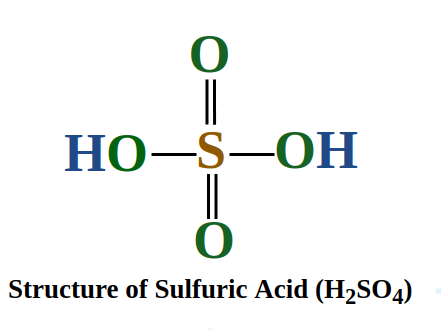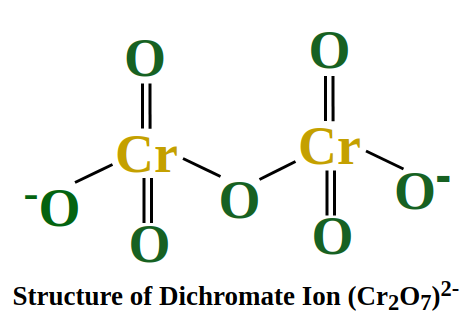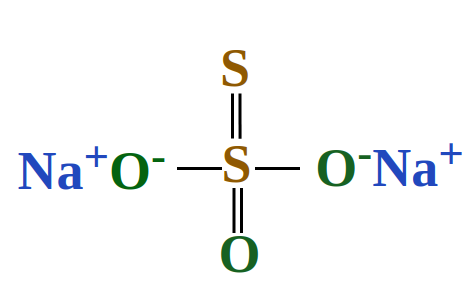Open In App
Related Articles
• CBSE Class 11 Chemistry Notes

# Oxidation Number

Oxidation Number is defined as the charge whether positive or negative gained by an atom of an element when they form bonds with atoms of other elements either by sharing or transferring electrons. The charge that appears as an oxidation number is basically the number of electrons lost, gained or shared by the atom during the formation of a bond with atoms of another element in a molecule. The oxidation number of an atom is often referred to as its oxidation state.

Oxidation number is generally assigned to atoms in the reaction in which they undergo oxidation and reduction. Such a reaction in which atoms either get oxidised or get reduced is called a redox reaction. The word redox is an acronym of two words reduction and oxidation.

## Oxidation Number Definition

The oxidation number of an atom is defined as the charge acquired by the atom when they form ionic bonds with atoms of another element i.e. heteroatoms in a molecule. Oxidation number can be positive, negative, zero or fraction depending upon the situation. A point to be noted here is that we always assume to be ionic for the calculation of oxidation number. Since every molecule possesses partial ionic and partially covalent character we assume ionic character for ease of calculation. In a molecule, it is assumed that more electronegative element gain electrons in whole numbers irrespective of sharing and less electronegative elements lose electrons in whole numbers thus making the bond ionic hypothetically. We can understand this by the following example in the case of Carbon monoxide(CO).

### Oxidation and Reduction

The definitions for oxidation and reduction are given as follows:

Oxidation: The loss of electrons or a rise in the positive oxidation state or decrease in the negative oxidation state of an atom, an ion, or specific atoms in a molecule is referred to as oxidation.

Reduction: The gain of electrons or a drop in the positive oxidation state or increase in the negative oxidation state of an atom, an ion, or specific atoms in a molecule is referred to as reduction (a reduction in the oxidation state).

## Oxidation Number of C in CO

In Carbon Monoxide we know that bond involved between the Carbon atom and the Oxygen atom is covalent but oxygen being more electronegative gain electron from the carbon atom in whole numbers. Since oxygen can gain a maximum of two electrons so its oxidation number will be -2. Now assume the oxidation state of Carbon in CO to be X Since CO is an electrically neutral molecule i.e. its overall charge is zero hence the sum of oxidation numbers of C and O will be zero i.e.

⇒X + (-2) = 0

⇒X – 2 = 0

⇒X = +2

Hence the oxidation state of the carbon atom in carbon monoxide is +2.

## Method to Calculate Oxidation Number

The oxidation number of an atom is the number of electrons lost or gained to form bonds with heteroatoms in a particular molecule. The molecule can be neutral i.e. its overall charge is zero or may be charged i.e. it has some overall positive or negative charge. To find the oxidation number of an atom we need to follow the following mentioned steps:

Step 1: Assume the oxidation number of the atom to be X which you need to calculate.

Step 2:Mention the oxidation state of other bonded atoms and multiply it with the number of such atoms present in one molecule

Step 3: Write the oxidation number of all the atoms in the molecule in a linear sum format and equate it to the overall charge of the molecule.

Step 4: Solve for X.

The calculation of oxidation number can be understood by the following examples. The atom in a molecule can exhibit only one oxidation state or more than one depending upon how many times they appear. Hence we will understand these in two different cases.

## Calculation of Oxidation Number of Atom

We can learn how to calculate the oxidation number of atoms with the examples discussed below,

Example 1: Calculate the Oxidation Number of Sulphur in H2SO4Solution:

Step 1: Assume the oxidation number of sulphur to be x

Step 2: The oxidation number for Hydrogen is +1 and for O is -2.

Step 3: Since the overall charge on the molecule is 0, therefore 2(+1) + X + 4(-2) = 0

Step 4: 2 + X – 8 = 0 ⇒ X – 6 = 0 ⇒ X = +6

Hence, the oxidation number of Sulphur in H2SO4 is +6

Example 2: Calculate the Oxidation Number of Chromium in Cr2O72-Solution:

Step 1: Assume the oxidation number for Chromium be X

Step 2: The oxidation number for oxygen is -2

Step 3: Since the molecule has an overall charge of -2 the equation can be written as 2X + 7(-2)=-2

Step 4: 2X – 14 = -2 ⇒ 2X = +12 ⇒ X = +6

Hence the oxidation number of chromium in Cr2O72- is +6.

It should be noted that in one molecule there can be different oxidation numbers for the same atom. This can be understood from the following example

### Calculation of Multiple Oxidation Numbers of an atom in a molecule

Example: Calculate the Oxidation Number of Nitrogen in Ammonium Nitrate i.e. NH4NO3

Solution:

Step 1: In this case, we will split the molecule into two ions Ammonium ion (NH4+) and Nitrate ion (NO3)

Step 2: We will find the oxidation number of Nitrogen in each ion. Assume the oxidation number for Nitrogen to be X in each case

Step 3: For the Ammonium ion, since the molecule has an overall +1 charge, hence equation can be written as X + 4(+1) = +1

Step 4: Solving the equation X + 4 = 1 ⇒ X = -3

Step 5: In the case of nitrate ion, the oxidation number for oxygen is -2 and the molecule has an overall charge of -1.

Step 6: Hence equation, in this case, will be X + 3(-2) = -1

Step 7: Solving the equation we get X – 6 = -1 ⇒ X = +5

Hence the oxidation number of Nitrogen in ammonium nitrate is -3 and +5

In the above examples, we saw that some atoms have fixed oxidation numbers. To understand why some atoms have fixed atomic numbers we need to look at the following mentioned rules of oxidation number.

## Rules for Assigning Oxidation Numbers

There are following rules to assign constant oxidation numbers to atoms of an element

Rule 1: The total of all the atoms in a molecule’s oxidation numbers equals zero.

e.g. In KMnO4, the oxidation number of K is +1, the oxidation number of Mn is +7 and the oxidation number of oxygen is −2.

Rule 2: An atom’s oxidation number is always 0 in its most basic form.

e.g. The oxidation number of H, O, N, P, S, Se, Cu, Ag in their element forms is H2, O2, N2, P4, S8, Se8, Cu, Ag respectively, is zero.

Rule 3: Alkali metals (Li, Na, K, Rb, Cs) have an oxidation number of +1 in their compounds.

e.g. In NaCl, the oxidation number of Na is +1.

Rule 4: Alkaline earth metals (Be, Mg, Ca, Sr, Ba) usually have a +2 oxidation number in their compounds.

e.g. The oxidation number of Mg in MgO is +2.

Rule 5: Except in metal hydrides, the oxidation number of H in its compound is always +1.

e.g. In HCl, the oxidation number of H is +1 and in NaH (Sodium hydride), the oxidation number of H is −1.

Rule 6: The fluorine oxidation number is 1 in all its compounds.

e.g. In NaF, the oxidation number of F is −1.

Rule 7: The charge of an ionic compound is equal to the sum of all oxidation states of all the atoms in the compound.

e.g. In SO42- the oxidation number of Sulphur is +6. The oxidation number of oxygen is −2. Hence the sum is +6+4(-2) = 6-8 = -2.

Rule 8: Except for oxygen and fluorine, the maximum oxidation number of any element is equal to its group number.

e.g. The oxidation number of sulphur in H2S2O8, K2S2O8, S2O–28 and H2SO5 is +6 due to the presence of a peroxide bond.

Rule 9: It’s possible that not all atoms of the same element have the same oxidation number in certain compounds. We get the average result when we compute the oxidation number for that element in such components.

e.g. One sulphur atom in Na2S2O3 has an oxidation number of +6, whereas the other sulphur atoms have an oxidation number of -2. As a result, the average sulphur oxidation state number in Na2S2O3 is +2.Rule 10: Carbon in organic molecules can have any oxidation number between -4 and +4.

e.g. in HCHO, the oxidation number of carbon is zero.

Rule 11: The common oxidation number of an element is equivalent to its group number from IA to IV A. The formula (Group number –8) gives the common oxidation number of any element from V A to VIII-A.

e.g.

• Oxidation number of I A group elements = +1.
• Oxidation number of II A group elements = +2.
• Oxidation number of III A group elements = +3.
• Oxidation number of IV A group elements = +4.
• Oxidation number of V A group elements = –3.
• Oxidation number of VI A group elements = –2.
• Oxidation number of VII A group elements = –1.
• Oxidation number of VIII A group elements = 0.

Rule 12: C, N, P, and S have oxidation numbers of 4,–3,–3 and –2 in all carbides, nitrides, phosphides, and sulphides, respectively.

e.g. In Mg3N2, the oxidation number of Nitrogen is −3.

Rule 13: In all Metal carbonyls, the oxidation number of metals is zero.

e.g. In Ni(CO)4, the oxidation number of Ni is zero.

Rule 14: Except for peroxide, superoxides, oxyfluorides, and ozonides, oxygen has an oxidation number of -2 in most of its oxides.

e.g. In Na2O, the oxidation number of O is −2.

### Exceptional Cases for Oxidation Number of Oxygen

• Peroxides: The oxidation number of oxygen in peroxides is 1.  Examples, are H2O2, and Na2O2.
• Oxidation number of oxygen in fluorine compounds is +2. Examples are F2O or OF2, etc.
• Superoxides: The oxidation number of oxygen in superoxides is –1/2.
• Ozonides: Each oxygen atom in an ozonide has an oxidation number of –1/3.

## Calculation of Oxidation Number of Some Common Compounds

As of now, we know the basic rules to calculate the Oxidation Number, hence we will find the Oxidation Number of some common compounds which we come across frequently in chemistry. We will find the Oxidation Number of the following compounds:

• Potassium Permanganate (KMnO4)
• Sodium Carbonate (Na2CO3)
• Ammonium Nitrite (NH4NO2)
• Cyanide Ion (CN)
• Ammonia (NH3)

## Oxidation Number of Manganese in KMnO4

Solution:

Let the oxidation number of Manganese in KMnO4 be equal to X

The oxidation number of Potassium = +1

The oxidation number of oxygen is =  –2

⇒1 + (X) + 4×(–2)=0

⇒1 + X – 8 = 0

⇒X -7 = 0

⇒X = +7

The oxidation number of Manganese in KMnO4 is +7.

## Oxidation Number of Carbon in Na2CO3

Solution:

Oxidation number of oxygen is = –2

Oxidation number of Sodium = +1

Let the oxidation number of carbon be X

⇒2(+1) + X + 3(–2) = 0

⇒2 + X – 6 = 0

⇒X – 4 = 0

⇒X = +4

Therefore X = +4

Oxidation Number of Carbon in Na2CO3 is +4

## Oxidation Number of Nitrogen in Ammonium Nitrite

Solution:

Ammonium Nitrite is an ionic compound containing NH4+ and NO2 ions.

Oxidation number of nitrogen in NH4+

Let oxidation number of N atom is x

⇒ x + 4 = +1

⇒ x = 1 – 4 = -3

Oxidation number of nitrogen in NO2

Let oxidation number of Nitrogen atom be Y

Y + 2(-2) = -1

⇒ Y – 4 = -1

⇒ Y = -1 + 4 = +3

Thus, one atom of Nitrogen in Ammonium Nitrite is in the -3 oxidation state, while the other nitrogen atom is in the +3 oxidation state.

## Oxidation Number of Carbon in CN–

Solution:

Let Oxidation Number of C atom in CN be x, Oxidation Number for N atom is -3 and the overall charge on the molecule is -1.

⇒ x – 3 = -1

⇒ x = -1 + 3 = +2

Hence, Carbon atom has oxidation number of +2 in CN

## Oxidation Number of N in NH3

Solution:

Let Oxidation Number of N in NH3 be x and we know that Oxidation Number of H is +1.

⇒ x + 3(+1) = 0

⇒ x + 3 = 0

⇒ x = -3

Hence, the Oxidation Number of N atom in NH3 is -3.

## FAQs on Oxidation Number

### Q1: What is Oxidation Number?

The charge that appears on an atom of an element when it bonds with atoms of other elements in a molecule is called Oxidation Number.

### Q2: What is the Common Oxidation Number for Inert Gases?

Inert gases have an oxidation number of zero.

### Q3: What are the Rules for Oxidation Numbers?

The rules for oxidation numbers are:

1. The total of all the atoms in a molecule’s oxidation numbers equals zero.
2. An atom’s oxidation number in its most basic form is always zero.
3. In their compounds, the oxidation number of alkali metals (Li, Na ,K ,Rb ,Cs) is always +1.
4. In their compounds, the oxidation number of alkaline earth metals (Be ,Mg ,Ca ,Sr ,Ba) is always +2.
5. Except in metal hydrides, the oxidation number of H in its compound is always +1.
6. Fluorine has an oxidation number of 1 in all of its compounds.
7. Except for peroxide, super oxides, oxyfluoride, and ozonides, the oxidation number of oxygen in most of its oxides is -2.
8. Carbon in organic molecules can have any oxidation number between -4 and +4.

### Q4: What is the Oxidation Number of Mercury(Hg) in Amalgam?

Mercury amalgam has a zero oxidation number. Each element in an alloy or amalgam has an oxidation number of zero.

### Q5: What is the Oxidation Number of Oxygen in O3 and in MgO?

• Oxidation number of O in O3 = 0
• Oxidation number of O in MgO = –2